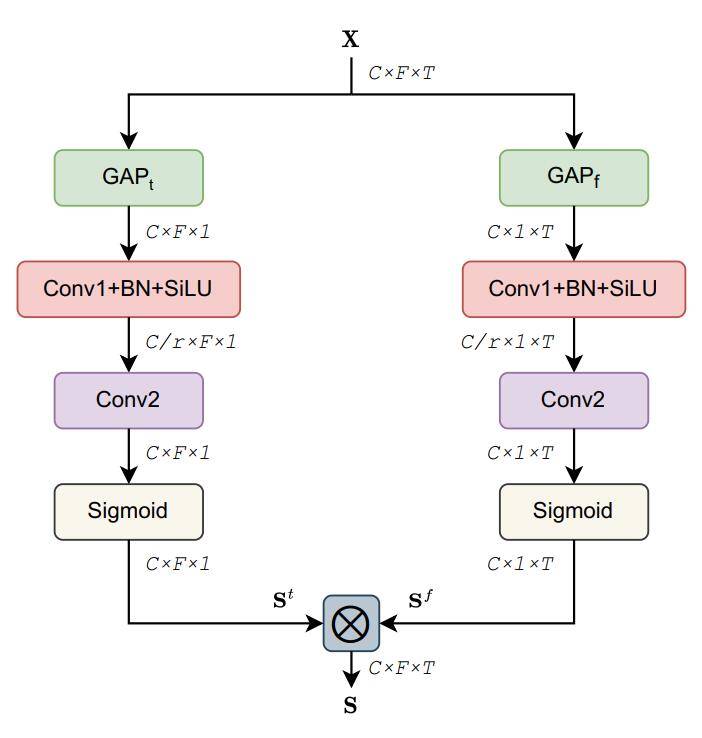# python中的不定量参数传递

*args 和 **kwargs 主要用于函数定义。其实这里写成什么样都无所谓，但是一般*args 和 **kwargs已经是约定俗成的写法，所以为了代码规范，大家一般都这样写。函数中的这样的形参意味着你可以将不定量的参数传递给一个函数。为什么要这样做呢？因为有时候我们并不确定需要传入哪些参数，所以这需要这样的写法。

## python中参数传递的方法

1. 位置传递

``````def fun(a,b,c):
return a+b*c
print(f(1,2,3)) #7
print(f(3,2,1)) #5
``````

这里每个实参和形参的位置严格的一一对应，一旦位置有所偏差就会造成不同的结果。

2. 关键字传递

``````def fun(a,b,c):
return a+b*c
print(f(a=1,b=2,c=3)) #7
print(f(c=3,2,a=1)) #7
``````

关键字传递是根据每个参数的名字传递参数,并不用遵守位置的对应关系。

3. 参数默认值

``````def fun(a,b,c=3):
return a+b*c
print(f(a=1,2)) #7
print(f(a=1,2,c=10)) #21
``````

有时在定义函数时会提前给参数赋一个默认值，如果调用函数时给参数赋其他值会覆盖默认值，否则函数会继续使用这个默认值。

• *类似于位置传参
• **类似于关键字传参
1. 包裹传递
`````` def func(*args):
print(type(args))
print(args)

func(1,4,6) #<class 'tuple'> (1, 4, 6)
func(5,6,7,1,2,3) #class 'tuple'> (5, 6, 7, 1, 2, 3)
``````

`````` def func(**kwargs ):
print (type(kwargs))
print (kwargs)

fun(a="2",b="1",c="11") # <class 'dict'> {'a': '2', 'b': '1', 'c': '11'}
``````

1. 解包裹

*和**同样也可以用于调用函数。

``````def func(a,b,c):
print a,b,c

args = (1,3,4)
func(*args) # 1,3,4

dict = {'a':1,'b':2,'c':3}
func(**dict) # 1,2,3
``````

## 参考资料

上一篇声纹识别论文阅读（四）

2022-10-21声纹识别论文阅读（三）

2022-10-07
目录